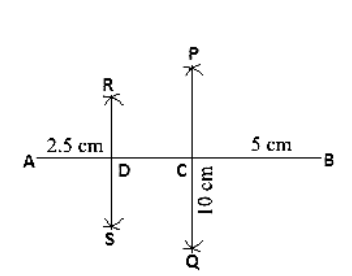# Draw a line segment of length 10 cm and bisect it. Further, bisect one of the equal parts and measure its length.

Question:

Draw a line segment of length 10 cm and bisect it. Further, bisect one of the equal parts and measure its length.

Solution:

Steps of construction:

1. Draw a line segment AB of length 10 cm.

2. Keeping A as center and radius more than half of AB draw arcs one on each side of AB.

3. Keeping B as center and same radius draw arcs cutting the previous arc at point P and Q respectively.

4. Join P and Q which intersects AB at C.

5. Keeping A as center and radius more than half of AC draw arcs on each side of AC.

6. Keeping C as center and the same radius draw arcs cutting the previous arcs at points R and S respectively.

7. Join points R and S which intersects AC at D.